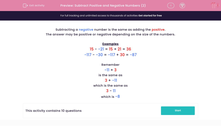# Subtract Positive and Negative Numbers (2)

In this worksheet, students subtract positive and negative numbers.This content is premium and exclusive to EdPlace subscribers.Key stage:  KS 2

Curriculum topic:   Maths and Numerical Reasoning

Curriculum subtopic:   Mixed Problems

Difficulty level:#### Worksheet Overview

Subtracting a negative number is the same as adding the positive.

The answer may be positive or negative depending on the size of the numbers.

Examples

15 - -21 = 15 + 21 = 36

-117 - -30 = -117 + 30 = -87

Remember

-11 + 3

is the same as

3 + -11

which is the same as

3 - 11

which is -8

### What is EdPlace?

We're your National Curriculum aligned online education content provider helping each child succeed in English, maths and science from year 1 to GCSE. With an EdPlace account you’ll be able to track and measure progress, helping each child achieve their best. We build confidence and attainment by personalising each child’s learning at a level that suits them.

Get startedTry an activity or get started for free